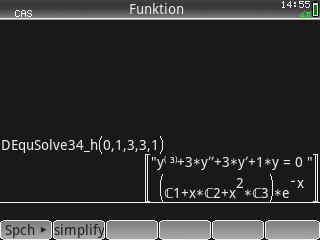## Differential Equation of 4th and 3rd Order, Part 1

Description:Finds the solution y(x) = y_hom + y_part to the differential equation (D.E.) of 4th order a*y^(4) + b*y''' + c*y'' + d*y' + e*y = g(x) or of 3rd order b*y''' + c*y'' + d*y' + e*y = g(x), where a = 0.
Filename:diffequat34part1.zip
ID:9231
Current version:1.03
Author:Claus Martin Dachselt
Size on calculator:15 KB
Platforms:Prime
User rating:Not yet rated (you must be logged in to vote)
Primary category:Math
Languages:ENG
File date:2020-12-02 08:32:37
Creation date:2020-12-02
Source code:Not included
Related files:Differential Equation of 4th and 3rd Order, Part 2
Screenshot: